﻿ 基于改进型BP神经网络的手部动作识别
«上一篇文章快速检索 高级检索

 智能系统学报2018, Vol. 13Issue (5): 848-854  DOI: 10.11992/tis.2017030180

### 引用本文YOU Bo, LI Zhongjie, HUANG Ling. Hand-motion recognition based on improved BP neural network[J]. CAAI Transactions on Intelligent Systems, 2018, 13(5): 848-854. DOI: 10.11992/tis.201703018.### 文章历史

Hand-motion recognition based on improved BP neural network
YOU Bo, LI Zhongjie, HUANG Ling
School of Automation, Harbin University of Science and Technology, Harbin 150080, China
Abstract: To achieve accurate pattern recognition of hand motions, in this study, we first denoised collected electromyogram (EMG) signals, and then used a variance algorithm in the time domain to extract features from the collected signals. After normalizing the characteristic signal, in the experiment, we found that the general BP neural network classifier has a slow learning rate, poor generalization ability, and large differences in its accuracy of recognizing diverse motions. To address the above problems, we propose an improved BP neural network that processes its input data by artificially increasing the dimensions. It then theoretically determines the reason for the slow network learning rate, and introduces a cross-entropy cost function to regularize it, thereby improving the network's generalization ability and increasing its reaction speed. Experimental results show that the improved BP neural network has a better learning speed, generalization ability, and accuracy in hand motion classification than the ordinary neural network, with an average recognition accuracy of 94.34%.
Key words: BP neural network    sEMG signal    cross entropy    hand-motion recognition    feature extraction    regularization    machine learning    pattern recognition

BP神经网络作为分类器，其应用同样广泛。例如，王尔申等将BP神经网络和基本粒子滤波算法进行有机结合有效地改善了滤波性能；赵漫丹等采用BP神经网络对AR模型的参数特征进行模式识别。近期国内外很多科研人员在研究深度神经网络在模式识别上的应用，识别准确率有了很大的提升。但深度神经网络训练时间长、计算量大、对硬件要求高，不利于识别系统的快速反应。因此，本文并未采用深度神经网络，而是对普通BP神经网络加以改进,以保证动作识别的快速性和准确性。

1 动作信号的模式识别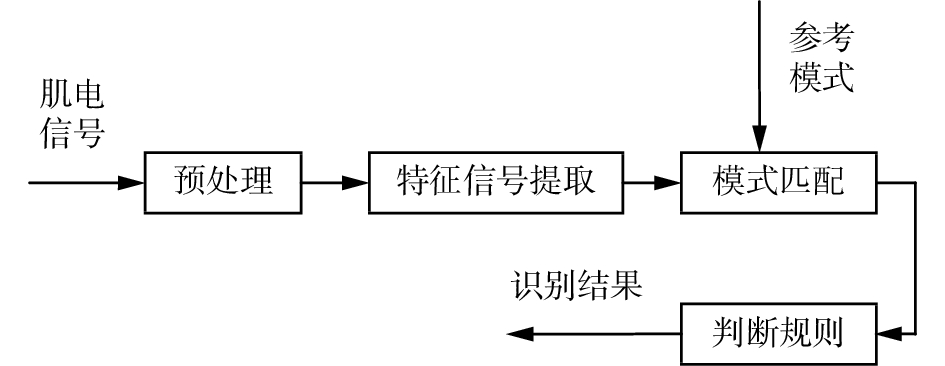Download: 图 1 动作识别流程 Fig. 1 Motion recognition process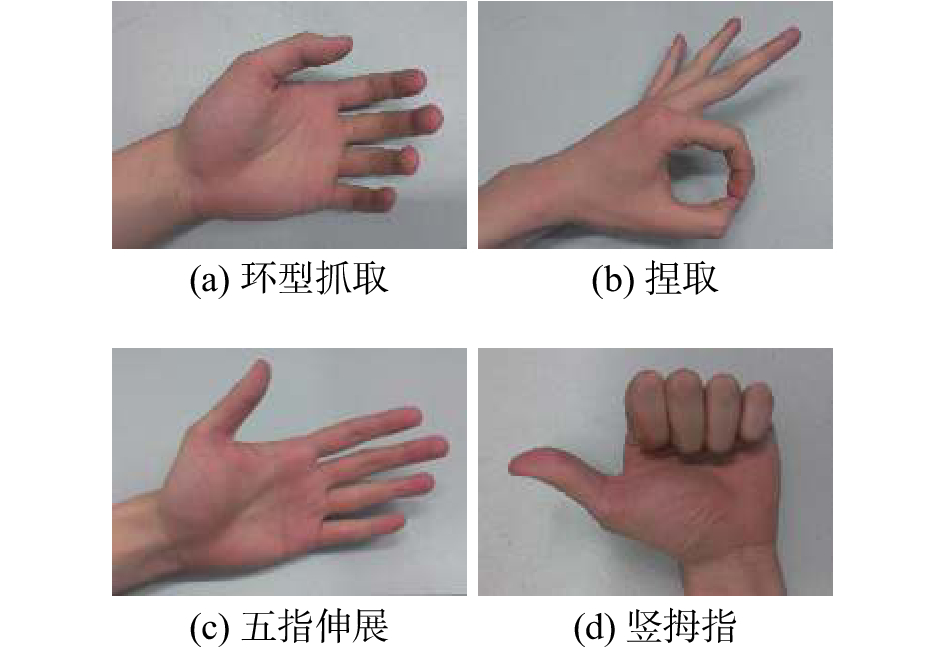Download: 图 2 4种手部基本动作 Fig. 2 Four basic hand motions
2 改进型BP神经网络的设计

BP神经网络的英文全称为back-propagation neural network，即反向传播神经网络。在隐含层神经元个数可以随意调整的前提下，其可以逼近任意的非线性映射，且具有一定的容错能力，故适合作为肌电信号的分类器。BP神经网络包含输入层、隐含层和输出层3部分，具体网络架构如图3所示。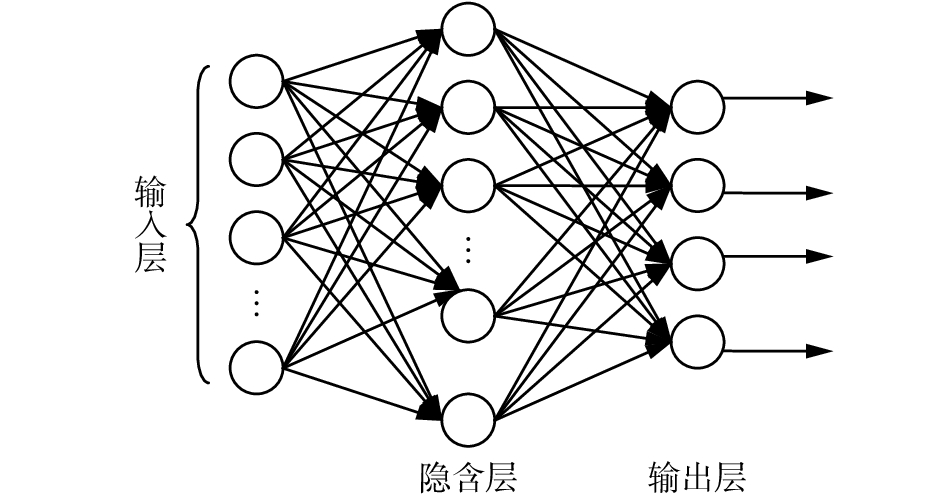Download: 图 3 BP神经网络架构示意 Fig. 3 Diagram of BP neural network framework

1) 输入层和输出层节点的设计

2) 隐含层节点数的设计

 \left\{ \begin{aligned} & l < \sqrt {(m + n)} + a \\ & l < n - 1 \\ & l = {\log _2}n \\ \end{aligned} \right. (1)

3) 初始值的选取

4) 代价函数的选择与改进

 $C = \frac{{{{\left( {{y_0} - y} \right)}^2}}}{2}$ (2)

 $\frac{{\partial C}}{{\partial \omega }} = \left( {y - {y_0}} \right){\sigma '}\left( z \right)x$ (3)
 $\frac{{\partial C}}{{\partial b}} = \left( {y - {y_0}} \right){\sigma '}\left( z \right)$ (4)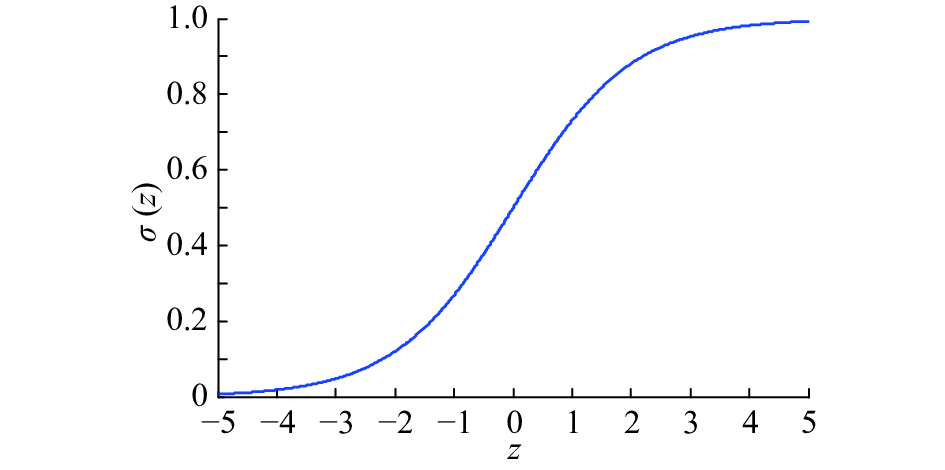Download: 图 4 Sigmoid函数 Fig. 4 Sigmoid function image

 $C = - \frac{1}{n}\sum\limits_x {\left[ {{y_0}\ln y + \left( {1 - {y_0}} \right)\ln \left( {1 - y} \right)} \right]}$ (5)

 \begin{aligned} \frac{{\partial C}}{{\partial \omega }} = & - \frac{1}{n}\sum\limits_x {\left[ {\frac{{{y_0}}}{{\sigma \left( z \right)}} - \frac{{\left( {1 - {y_0}} \right)}}{{1 - \sigma \left( z \right)}}} \right]} \frac{{\partial \sigma }}{{\partial \omega }} = \\ & \frac{1}{n}\sum\limits_x {x\left( {\sigma \left( z \right) - {y_0}} \right)} \\ \end{aligned} (6)

5) 对代价函数进行正则化处理

 ${C_0} = - \frac{1}{n}\sum\limits_x {\left[ {{y_0}\ln y + \left( {1 - {y_0}} \right)\ln \left( {1 - y} \right) + \frac{\lambda }{{2n}}\sum\limits_\omega {{\omega ^2}} } \right]}$

 $b = b - \eta \frac{{\partial {C_0}}}{{\partial b}}$
 $\begin{gathered} \omega = \omega - \eta \frac{{\partial {C_0}}}{{\partial \omega }} - \frac{{\eta \lambda }}{n}\omega = \left( {1 - \frac{{\eta \lambda }}{n}} \right)\omega - \eta \frac{{\partial {C_0}}}{{\partial \omega }} \\ \end{gathered}$

3 实验设计与仿真结果分析 3.1 肌电信号的采集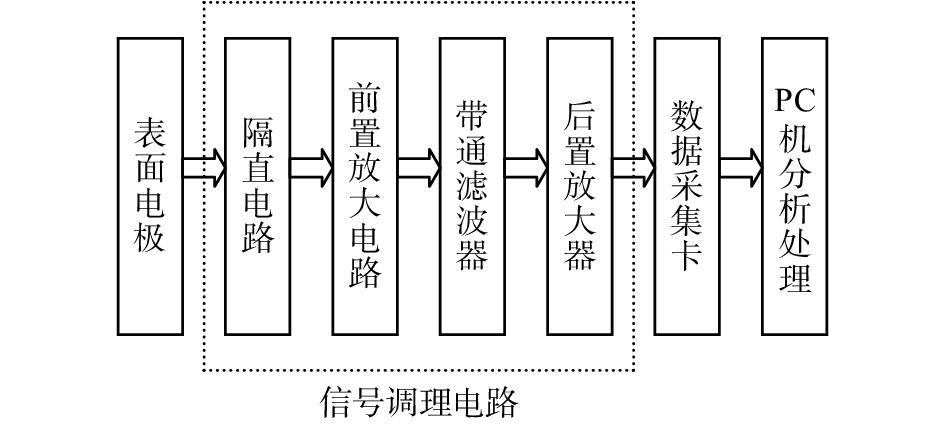Download: 图 5 表面肌电信号采集流程图 Fig. 5 sEMG signal acquisition flow chart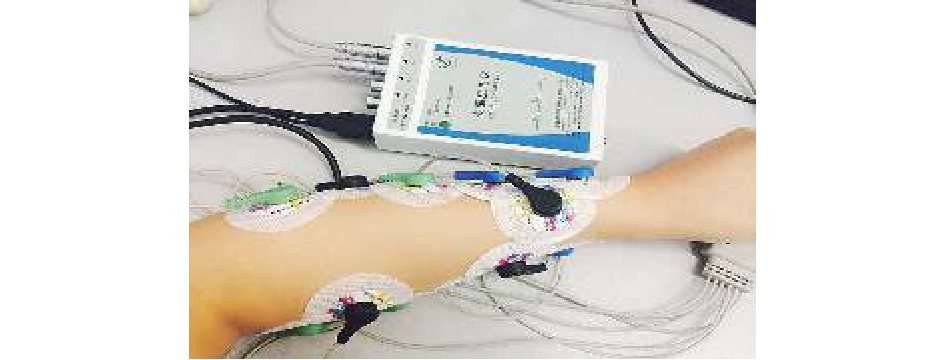Download: 图 6 表面肌电信号采集系统实物图 Fig. 6 sEMG signal acquisition system physical map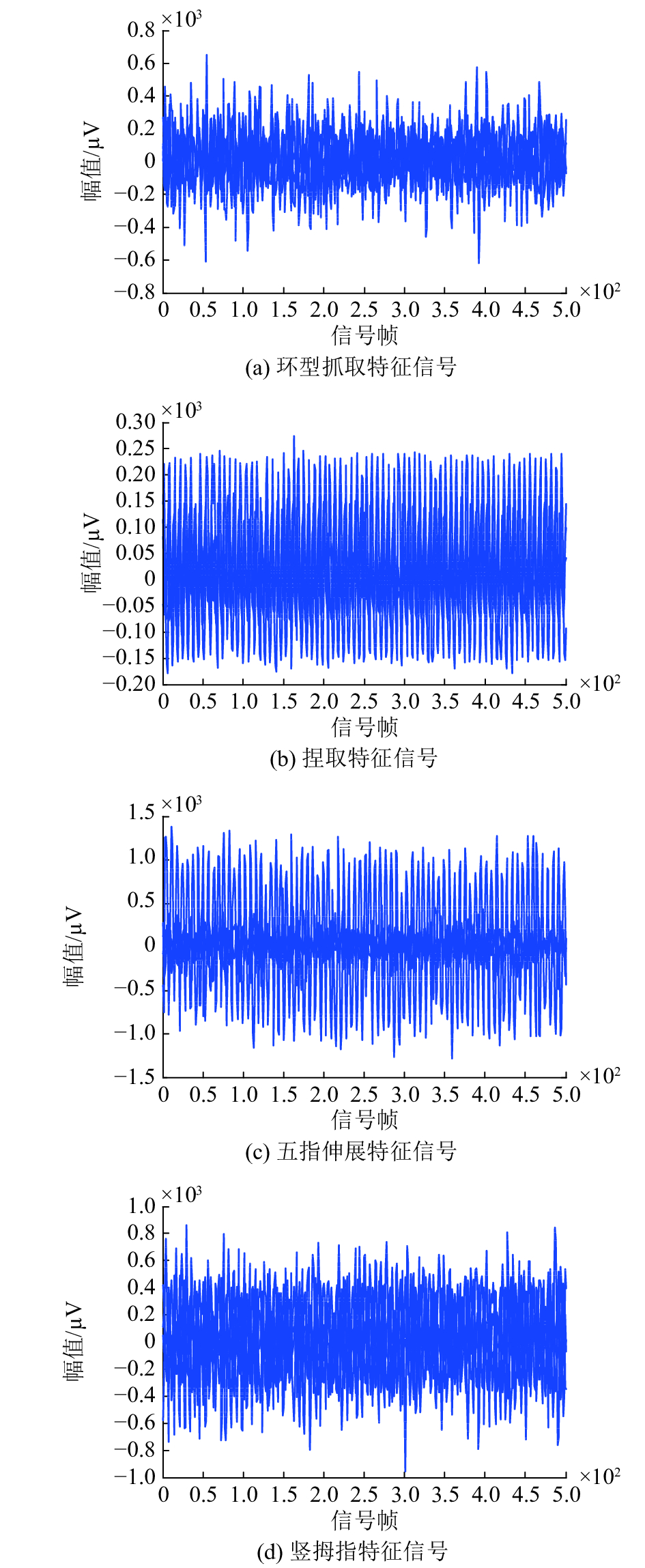Download: 图 7 4种手部动作肌电特征信号 Fig. 7 Four kinds of hand-motion EMG signals
3.2 肌电信号的特征提取

 ${\rm{VAR}} = \frac{1}{{N - 1}}\sum\limits_{i = 1}^N {({x_i}} - \mu {)^2}$

 ${\rm{VAR}} = \frac{1}{{N - 1}}\sum\limits_{i = 1}^N {x_i^2}$
3.3 数据处理及仿真实验结果分析

1)最大最小法

 ${x_k} = \frac{{{x_k} - {x_{\min }}}}{{{x_{\max }} - {x_{\min }}}}$

2)平均数方差法

 ${x_k} = \frac{{{x_k} - \bar x}}{{{x_{\operatorname{var} }}}}$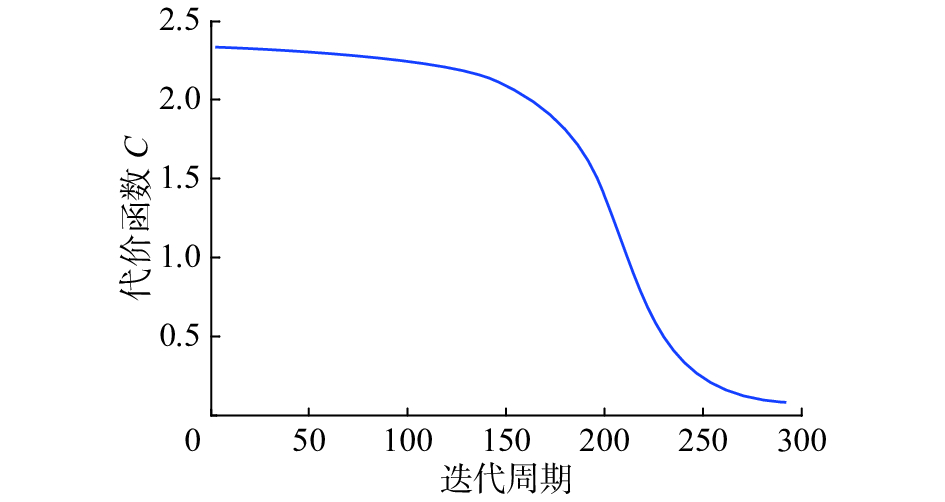Download: 图 8 普通BP网络代价函数曲线 Fig. 8 Cost function curve of ordinary BP network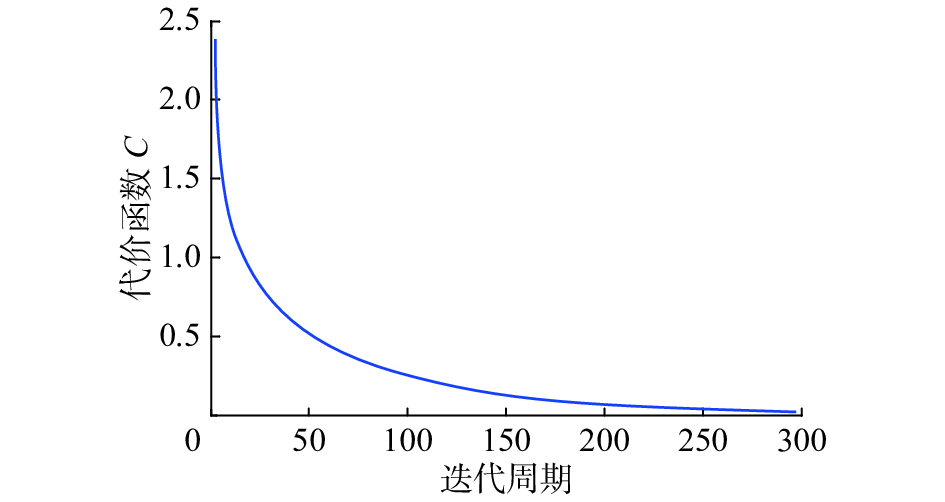Download: 图 9 改进型BP网络代价函数曲线 Fig. 9 Cost function curve of modified BP network

BP神经网络分类算法程序具体执行流程如图10所示。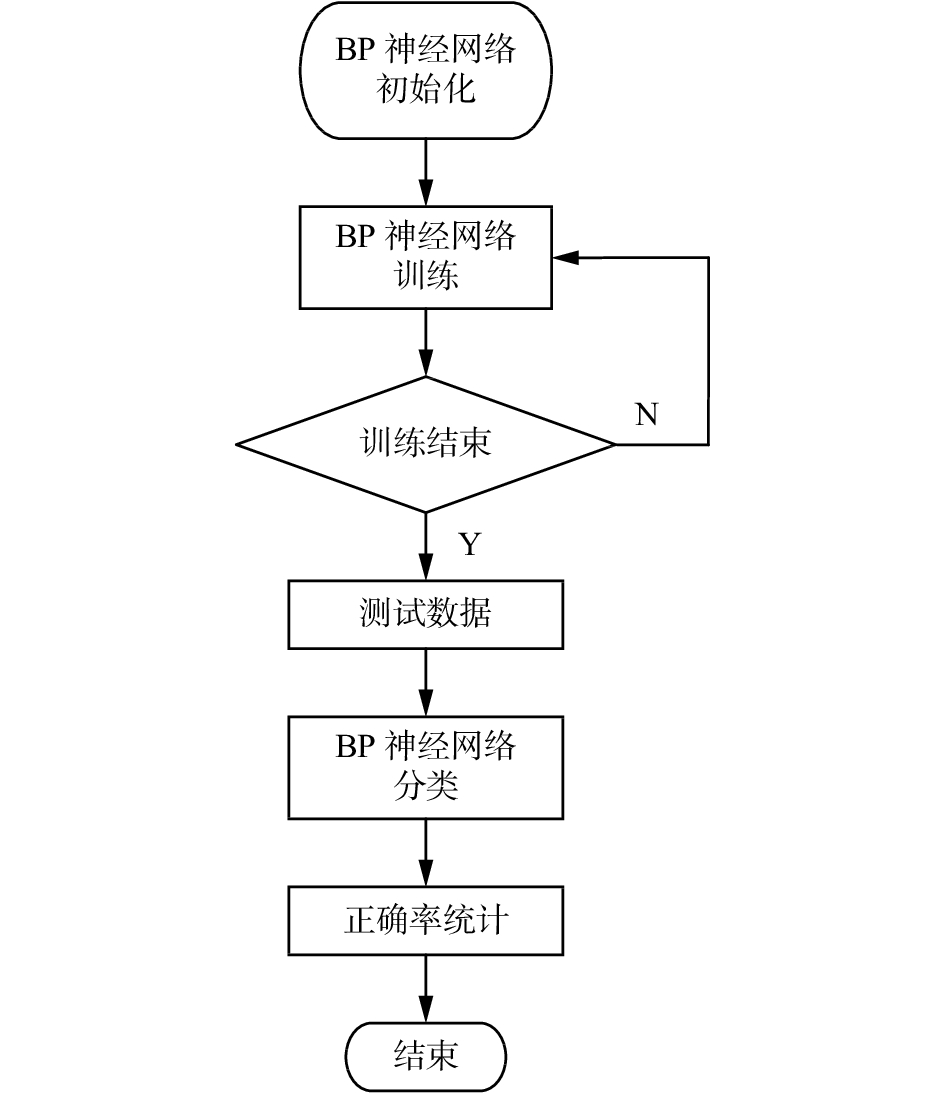Download: 图 10 程序执行流程图 Fig. 10 Program execution flowchart

 $\text{正确率} = \frac{{N - {N_{{\rm{error}}}}}}{N} \times 100\text{%}$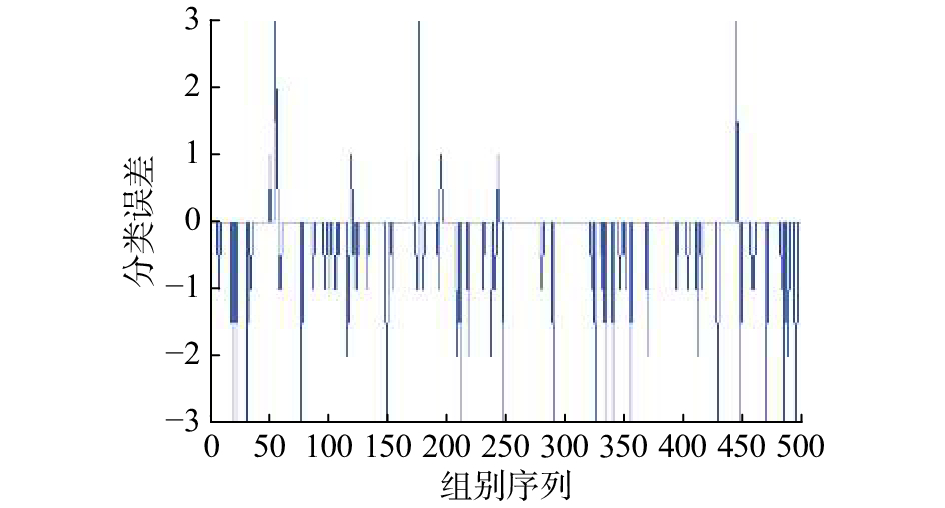Download: 图 11 普通BP神经网络分类误差 Fig. 11 Classification error of ordinary BP neural network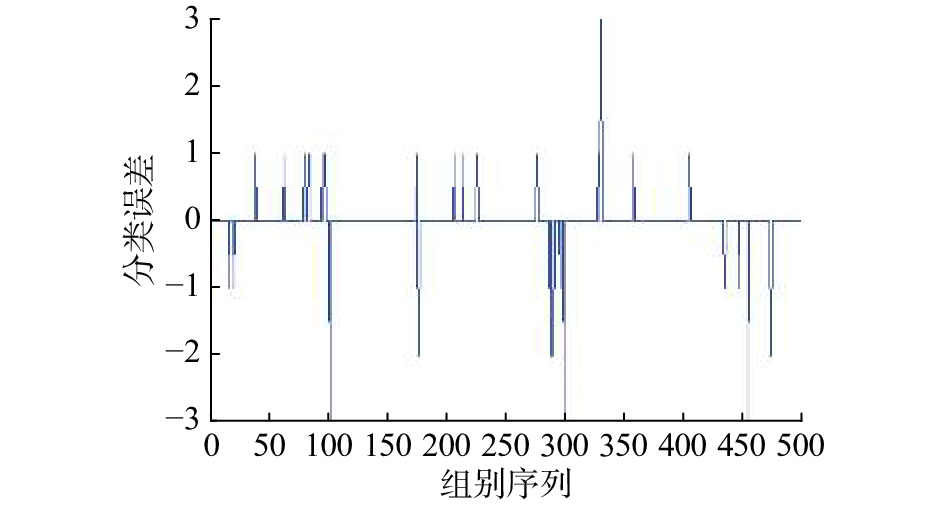Download: 图 12 改进型BP神经网络分类误差 Fig. 12 Classification error of modified BP neural network表 1 识别准确率统计结果 Tab.1 Recognition accuracy statistics表 2 动作识别耗时结果 Tab.2 Action recognition time-consumption results
4 结论

1)通过引入交叉熵代价函数，BP神经网络的学习速率得到了有效的提升,经少量迭代后代价函数值便迅速下降；

2)将输入端数据进行人工升维处理并将代价函数正则化后，网络的过度拟合现象得到了控制，泛化能力增强，动作识别准确率也得到了有效的提升。

  尤波, 杨文元. 基于小波理论的sEMG的处理方法[J]. 自动化技术与应用, 2012, 31(2): 55-57, 61. YOU Bo, YANG Wenyuan. The processing method about sEMG based on wavelet theory[J]. Techniques of automation and applications, 2012, 31(2): 55-57, 61. DOI:10.3969/j.issn.1003-7241.2012.02.014 (0)  YOU Bo, ZHOU Lina, HUANG Ling. The design of a recognition system for multi-function electrical rubber hand[J]. Transactions of the institute of measurement and control, 2016, 38(11): 1298-1310. DOI:10.1177/0142331215585154 (0)  谢平, 魏秀利, 杜义浩, 等. 基于自排序熵的表面肌电信号特征提取方法[J]. 模式识别与人工智能, 2014, 27(6): 496-501. XIE Ping, WEI Xiuli, DU Yihao, et al. Feature extraction method of sEMG based on auto permutation entropy[J]. Pattern recognition and artificial intelligence, 2014, 27(6): 496-501. DOI:10.3969/j.issn.1003-6059.2014.06.003 (0)  邱青菊, 朱向阳. 基于双谱分析表面肌电信号特征的提取与模式识别[J]. 中国组织工程研究与临床康复, 2010, 14(4): 645-648. QIU Qingju, ZHU Xiangyang. Feature extraction and pattern recognization of surface electromyography signal based on bispectrum analysis[J]. Journal of clinical rehabilitative tissue engineering research, 2010, 14(4): 645-648. (0)  张启忠, 席旭刚, 罗志增. 基于非线性特征的表面肌电信号模式识别方法[J]. 电子与信息学报, 2013, 35(9): 2054-2058. ZHANG Qizhong, XI Xugang, LUO Zhizeng. A pattern recognition method for surface electromyography based on nonlinear features[J]. Journal of electronics & information technology, 2013, 35(9): 2054-2058. (0)  NAIK G R, KUMAR D K, JAYADEVA J. Twin SVM for gesture classification using the surface electromyogram[J]. IEEE transactions on information technology in biomedicine-special section on affective and pervasive computing for healthcare, 2010, 14(2): 301-308. (0)  CHEN Y, ZHOU Y, CHENG X. Surface EMG based upper limb motion recognition in real-time[J]. Journal of computational information systems, 2013, 9(23): 9549-9556. (0)  席旭刚, 李仲宁, 罗志增. 基于相关性分析和支持向量机的手部肌电信号动作识别[J]. 电子与信息学报, 2008, 30(10): 2315-2319. XI Xugang, LI Zhongning, LUO Zhizeng. SEMG movement pattern recognition of hand based on correlation analysis and SVM[J]. Journal of electronics & information technology, 2008, 30(10): 2315-2319. (0)  LI Yang, TIAN Yantao, CHEN Wanzhong. sEMG pattern recognition based on GRNN and Adaboost[C]//International Conference on Electronics, Communications and Control. Ningbo, China, 2011: 1661–1664. (0)  张毅, 许新丽, 罗元. 基于在线SVM的自适应sEMG人机交互系统[J]. 华中科技大学学报: 自然科学版, 2013, 41(4): 75-79. ZHANG Yi, XU Xinli, LUO Yuan. Adaptive myoelectric human-machine interface systems using online support vector machines[J]. Journal of huazhong university of science & technology: nature science edition, 2013, 41(4): 75-79. (0)  KHEZRI M, JAHED M. A neuro–fuzzy inference system for sEMG-based identification of hand motion commands[J]. IEEE transactions on industrial electronics, 2011, 58(5): 1952-1960. DOI:10.1109/TIE.2010.2053334 (0)  NAIK G R, AL-TIMEMY A H, NGUYEN H T. Transradial amputee gesture classification using an optimal number of sEMG sensors: an approach using ICA clustering[J]. IEEE transactions on neural systems and rehabilitation engineering, 2016, 24(8): 837-846. DOI:10.1109/TNSRE.2015.2478138 (0)  KAINZ O, CYMBALÁK D, KARDOŠ S, et al. Low-cost assistive device for hand gesture recognition using sEMG[C]//Proceedings Volume 10011, First International Workshop on Pattern Recognition. Tokyo, Japan, 2016: 100111B. (0)  林海波, 王浩, 张毅, 等. 改进高斯核函数的人体姿态分析与识别[J]. 智能系统学报, 2015, 10(3): 436-441. LIN Haibo, WANG Hao, ZHANG Yi,et al. Human postures recognition based on the improved Gauss kernel function[J]. CAAI transactions on intelligent systems, 2015, 10(3): 436-441. (0)  杨帅. 基于AdaBoost算法的手部动作表面肌电信号分类方法研究[D]. 长春: 吉林大学, 2015: 35–57. YANG Shuai. Research of surface EMG classification of hand movement based on AdaBoost algorithm[D]. Changchun, China: Jilin University, 2015: 35–57. (0)  王尔申, 李兴凯, 庞涛. 基于BP神经网络的粒子滤波算法[J]. 智能系统学报, 2014, 9(6): 709-713. WANG Ershen, LI Xingkai, PANG Tao. A particle filtering algorithm based on the BP neural network[J]. CAAI transactions on intelligent systems, 2014, 9(6): 709-713. (0)  赵漫丹, 李东旭, 范才智, 等. 基于肌电信号层级分类的手部动作识别方法[J]. 北京生物医学工程, 2014, 33(5): 490-496. ZHAO Mandan, LI Dongxu, FAN Caizhi, et al. A method of hand movement pattern recognition based on sEMG hierarchical classification[J]. Beijing biomedical engineering, 2014, 33(5): 490-496. DOI:10.3969/j.issn.1002-3208.2014.05.10 (0)  葛哲学, 孙志强. 神经网络理论与MATLAB R2007实现[M]. 北京, 电子工业出版社, 2007: 108–112. (0)  吕国豪, 罗四维, 黄雅平, 等. 基于卷积神经网络的正则化方法[J]. 计算机研究与发展, 2014, 51(9): 1891-1900. LÜ Guohao, LUO Siwei, HUANG Yaping, et al. A novel regularization method based on convolution neural network[J]. Journal of computer research and development, 2014, 51(9): 1891-1900. (0)  赵谦, 孟德宇, 徐宗本. L1/2正则化Logistic回归[J]. 模式识别与人工智能, 2012, 25(5): 721-728. ZHAO Qian, MENG Deyu, XU Zongben. L1/2 regularized Logistic regression[J]. Pattern recognition and artificial intelligence, 2012, 25(5): 721-728. DOI:10.3969/j.issn.1003-6059.2012.05.001 (0)